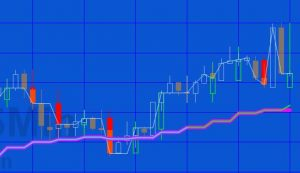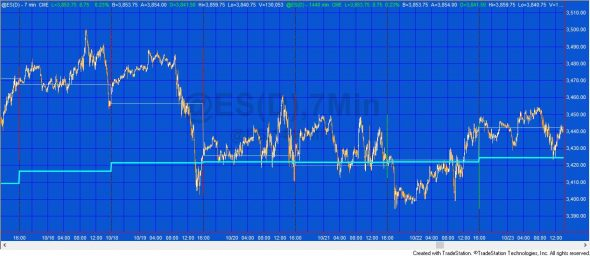# Tutorial 163 | Calculate an EMA using Price Series ProviderTutorial 163 applied to a 3 minute chart with a plot of a 15 period EMA as applied to a 10 minute chart

Tutorial 163 is designed to calculate the exponential moving average (EMA) of a longer time frame minute chart and plot it on a lower time frame chart using a price series provider (PSP). In this tutorial the EMA is also calculated by using data 2 in a simple calculation (the same as the Tradestation XAverage function)

The standard Tradestion EMA (XAverage) function using regular data starts calculating on the first bar of the chart. The next bar uses the value of the previous bar (which in turn used the values of the previous bar) and so on up to the current bar. The XAverage function uses the following code:

`XAverage = XAverage + SmoothingFactor * ( Price - XAverage );`

Instead of accumulating the values as was done in Tutorial 86, for every bar tutorial 163 counts back a number of bars and then does the calculation using the following (where XAv3 is a variable used to store the EMA):

```XAv3 = PSP.Close[BarsBack - 1]; // Set initial value
For Value1 = BarsBack - 1 downto 0
Begin
XAv3 = XAv3 + ( 2 / ( Length + 1 ) * ( PSP.Close[Value1] - XAv3 ) );
End;```

PSP objects and the equivalent price data streams are not tightly synchronized however the PSP does make sure that it does not expose information that is newer than the bar being calculated. This enables the above calculation.

This tutorial program is designed to plot a longer time frame EMA on a shorter time frame using minute bars. If applied to RadarScreen use the _Tutorial163-wo-data2 version of the program. When using RadarScreen make sure that you enter an appropriate number of bars in the “Load Additional Bars” setting.

It appears that with some time frame combinations that PSP is less well synchronized than others which causes some differences in the EMA calculation.

### Video walk-through of the tutorial codeTutorial 163 applied to a 7 minute chart plotting a 15 period EMA as applied to a 1440 minute chart